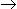# Aptitude - Numbers

### Exercise :: Numbers - General Questions

51.

476 ** 0 is divisible by both 3 and 11. The non-zero digits in the hundred's and ten's places are respectively:

 A. 7 and 4 B. 7 and 5 C. 8 and 5 D. None of these

Explanation:

Let the given number be 476 xy 0.

Then (4 + 7 + 6 + x + y + 0) = (17 + x + y) must be divisible by 3.

And, (0 + x + 7) - (y + 6 + 4) = (x - y -3) must be either 0 or 11.

x - y - 3 = 0y = x - 3

(17 + x + y) = (17 + x + x - 3) = (2x + 14)x= 2 or x = 8.x = 8 and y = 5.

52.

If the number 97215 * 6 is completely divisible by 11, then the smallest whole number in place of * will be:

 A. 3 B. 2 C. 1 D. 5 E. None of these

Explanation:

Given number = 97215x6

(6 + 5 + 2 + 9) - (x + 1 + 7) = (14 - x), which must be divisible by 11.x = 3

53.

(112 + 122 + 132 + ... + 202) = ?

 A. 385 B. 2485 C. 2870 D. 3255

Explanation:

(112 + 122 + 132 + ... + 202) = (12 + 22 + 32 + ... + 202) - (12 + 22 + 32 + ... + 102)Ref: (12 + 22 + 32 + ... + n2) = 1 n(n + 1)(2n + 1)6

 =20 x 21 x 41 - 10 x 11 x 216 6

= (2870 - 385)

= 2485.

54.

If the number 5 * 2 is divisible by 6, then * = ?

 A. 2 B. 3 C. 6 D. 7

Explanation:

6 = 3 x 2. Clearly, 5 * 2 is divisible by 2. Replace * by x.

Then, (5 + x + 2) must be divisible by 3. So, x = 2.

55.

Which of the following numbers will completely divide (4915 - 1) ?

 A. 8 B. 14 C. 46 D. 50

Explanation:

(xn - 1) will be divisibly by (x + 1) only when n is even.

(4915 - 1) = {(72)15 - 1} = (730 - 1), which is divisible by (7 +1), i.e., 8.

56.

 9 + 3 + 7 + 2 -9 + 1= ? 4 17 15

A.
 7 + 719 1020
B.
 9 + 817 1020
C.
 9 + 719 1020
D.
 7 + 817 1020
E. None of these

Explanation:

Given sum
 = 9 + 3 + 7 + 2 -9 + 14 17 15
 = (9 + 7 - 9) +3 + 2 - 14 17 15
 = 7 + 765 + 120 - 68 1020
 = 7 + 817 1020

57.1 - 1+1 - 2+1 - 3+ ... up to n terms = ? n n n

A.
 1 n 2
B.
 1 (n - 1) 2
C.
 1 n(n - 1) 2
D. None of these

Explanation:

Given sum
 = (1 + 1 + 1 + ... to n terms) -1 + 2 + 3 + ... to n termsn n n
 = n - n1 + 1[ Ref: nth terms = (n/n) = 1] 2 n
 = n - n + 1 2
 = 1 (n - 1) 2

58.

On dividing 2272 as well as 875 by 3-digit number N, we get the same remainder. The sum of the digits of N is:

 A. 10 B. 11 C. 12 D. 13

Explanation:

Clearly, (2272 - 875) = 1397, is exactly divisible by N.

Now, 1397 = 11 x 127The required 3-digit number is 127, the sum of whose digits is 10.

59.

A boy multiplied 987 by a certain number and obtained 559981 as his answer. If in the answer both 9 are wrong and the other digits are correct, then the correct answer would be:

 A. 553681 B. 555181 C. 555681 D. 556581

Explanation:

987 = 3 x 7 x 47

So, the required number must be divisible by each one of 3, 7, 47

553681(Sum of digits = 28, not divisible by 3)

555181(Sum of digits = 25, not divisible by 3)

555681 is divisible by 3, 7, 47.

60.

How many prime numbers are less than 50 ?

 A. 16 B. 15 C. 14 D. 18

Explanation:

Prime numbers less than 50 are:
2, 3, 5, 7, 11, 13, 17, 19, 23, 29, 31, 37, 41, 43, 47

Their number is 15